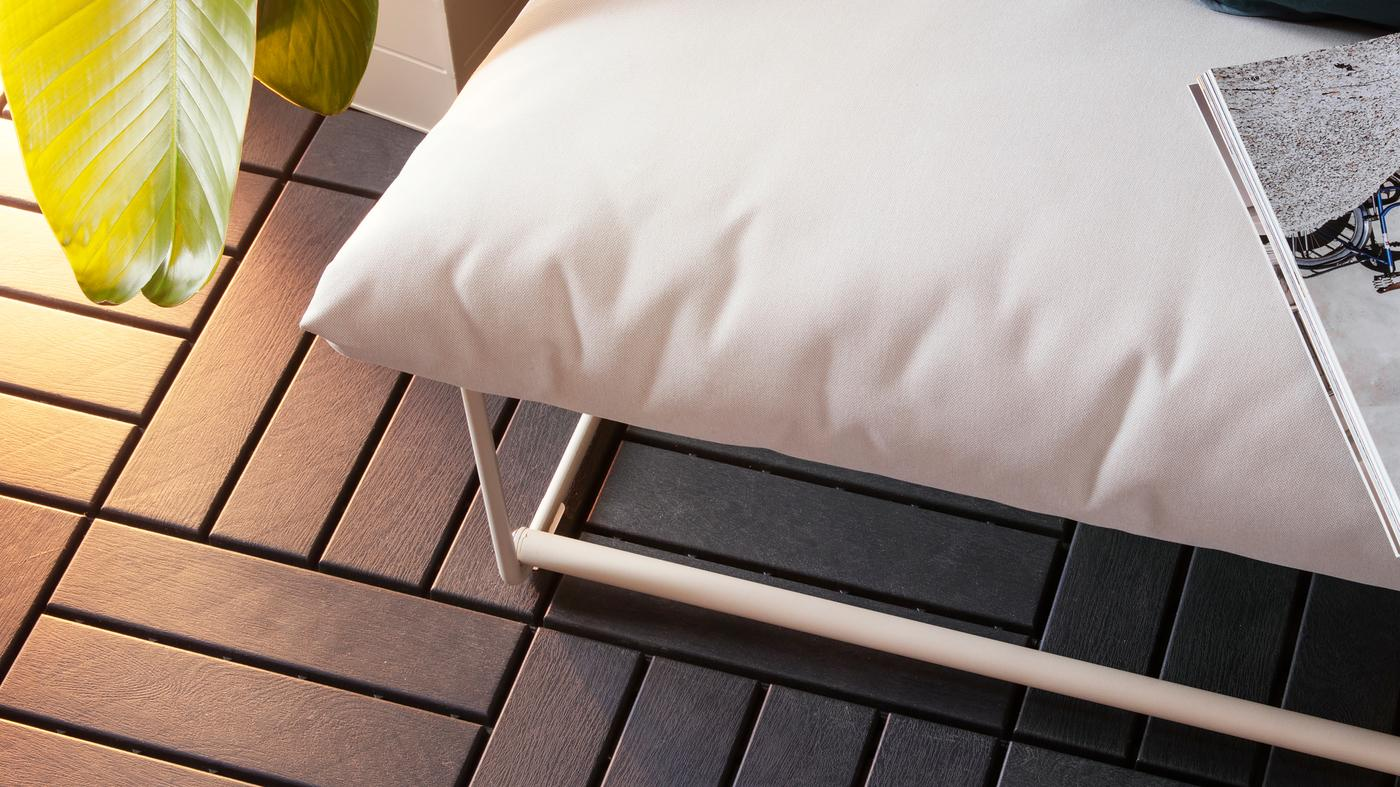Quickly create a personal look with a range of outdoor floor decking in different materials and colors. They click together, so it is easy to upgrade your outdoor flooring.## 1. Calculate the size of your space

To calculate your outdoor area, multiply the length by the width. For example, width 10 ft × 16 ft = 160 ft2. Calculate any areas to be subtracted from the total area, for example pillars or corners.

## 2. Calculate how many packages you need

Divide the sum by 8.72 and round up (to ensure enough floor decking). For example, 160 ft2 ÷ 8.72 = 18.34. Rounded up, that means you need 19 packages.